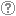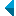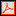# Iskanje po repozitorijuIskalni niz: išči po NaslovAvtorOpisKljučne besedePolno besediloLeto izida INALIIN NE išči po NaslovAvtorOpisKljučne besedePolno besediloLeto izida INALIIN NE išči po NaslovAvtorOpisKljučne besedePolno besediloLeto izida INALIIN NE išči po NaslovAvtorOpisKljučne besedePolno besediloLeto izida Vrsta gradiva: Vse vrste gradiv Habilitacijsko delo (m4) Višješolska diplomska naloga (m6) Specialistično delo (m3) Diplomsko delo * (dip) Magistrsko delo * (mag) Doktorska disertacija * (dok) Raziskovalni podatki ali korpus (data) * po starem in bolonjskem študiju Jezik: Vsi jezikiSlovenski jezikAngleški jezikNemški jezikHrvaški jezikSrbski jezikAfrikanski jezikAlbanski jezikArabski jezikArmenski jezikAzerbajdžanski jezikBolgarski jezikBosanski jezikČeški jezikČrnogorski jezikDanski jezikEstonski jezikFinski jezikFrancoski jezikGrški jezikGruzinski jezikIrski jezikItalijanski jezikJaponski jezikKatalonski jezikKazaški jezikKitajski jezikKorejski jezikLatinski jezikLatvijski jezikLitvanski jezikMadžarski jezikMakedonski jezikMalteški jezikNemški jezik (Avstrija)Nizozemski jezikNorveški jezikPoljski jezikPortugalski jezikRomunski jezikRuski jezikSlovanski jezik (ostali)Slovaški jezikSrbski jezik (cirilica)Španski jezikŠvedski jezikTurški jezikUkrajinski jezikNi določenVečjezičniNeznan jezikOstali Išči po: RUP    FAMNIT - Fakulteta za matematiko, naravoslovje in informacijske tehnologije    FHŠ - Fakulteta za humanistične študije    FM - Fakulteta za management    FTŠ Turistica - Fakulteta za turistične študije - Turistica    FVZ - Fakulteta za vede o zdravju    IAM - Inštitut Andrej Marušič    PEF - Pedagoška fakulteta    UPR - Univerza na Primorskem    ZUP - Založba Univerze na PrimorskemCOBISS    Fakulteta za humanistične študije, Koper    Fakulteta za management Koper in Pedagoška fakulteta Koper    Fakulteta za vede o zdravju, Izola    Knjižnica za tehniko, medicino in naravoslovje, Koper    Turistica, Portorož    Znanstveno-raziskovalno središče Koper Opcije: Prikaži samo zadetke s polnim besedilom Ponastavi

 1 - 10 / 12121.O nekaterih problemih, ki so povezani s Terwilligerjevimi algebrami in razdaljno-uravnoteženimi grafi : doktorska disertacijaBlas Fernández, 2023, doktorska disertacijaKljučne besede: distance-regularized vertex, pseudo-distance-regularized vertex, Terwilliger algebra, irreducible module, distance-balanced graph, nicely distance-balanced graph, strongly distance-balanced graphObjavljeno v RUP: 15.02.2023; Ogledov: 260; Prenosov: 8Povezava na polno besedilo 2.On bipartite graphs with exactly one irreducible T-module with endpoint 1, which is thinBlas Fernández, Štefko Miklavič, 2021, izvirni znanstveni članekKljučne besede: distance-biregular graph, Terwilliger algebra, irreducible moduleObjavljeno v RUP: 18.10.2021; Ogledov: 413; Prenosov: 26Povezava na polno besedilo 3.A combinatorial basis for Terwilliger algebra modules of a bipartite distance-regular graphMark MacLean, Safet Penjić, 2021, izvirni znanstveni članekKljučne besede: bipartite distance-regular graph, Terwilliger algebra, subconstituent algebra, T-modules of endpoint 2Objavljeno v RUP: 06.05.2021; Ogledov: 433; Prenosov: 28Povezava na polno besedilo 4.On the Terwilliger algebra of a certain family of bipartite distance-regular graphs with Delta_2 = 0Štefko Miklavič, Safet Penjić, 2020, izvirni znanstveni članekKljučne besede: distance-regular graphs, Terwilliger algebra, irreducible modulesObjavljeno v RUP: 17.08.2020; Ogledov: 926; Prenosov: 39Polno besedilo (420,51 KB) 5.On a certain class of 1-thin distance-regular graphsMark MacLean, Štefko Miklavič, 2020, izvirni znanstveni članekKljučne besede: distance-regular graph, Terwilliger algebra, subconstituent algebraObjavljeno v RUP: 20.07.2020; Ogledov: 944; Prenosov: 85Polno besedilo (379,60 KB) 6.O Terwilligerjevi algebri dvodelnih razdaljno-regularnih grafov : doktorska disertacijaSafet Penjić, 2019, doktorska disertacijaKljučne besede: bipartite distance-regular graph, Terwilliger algebra, subconstituent algebra, Q-polynomial property, equitable partitionObjavljeno v RUP: 04.06.2019; Ogledov: 1836; Prenosov: 26Povezava na polno besedilo 7.On the Terwilliger algebra of bipartite distance-regular graphs with $G_{i-1,i-1,1}(x, y, z) = alpha_i + beta_i G_{1,1,i%1}(x, y, z)$ : 31th Ljubljana - Leoben Graph Theory Seminar, September 3-4, 2018, LoebenSafet Penjić, 2018, prispevek na konferenci brez natisaKljučne besede: distance-regular graphs, Terwilliger algebra, irreducible modulesObjavljeno v RUP: 17.09.2018; Ogledov: 1303; Prenosov: 21Povezava na polno besedilo 8.An A-invariant subspace for bipartite distance-regular graphs with exactly two irreducible T-modules with endpoint 2, both thinMark MacLean, Štefko Miklavič, Safet Penjić, 2018, izvirni znanstveni članekKljučne besede: distance-regular graph, Terwilliger algebra, subconstituent algebra, A-invariant subspaceObjavljeno v RUP: 02.03.2018; Ogledov: 1628; Prenosov: 80Povezava na polno besedilo 9.On the Terwilliger algebra of bipartite distance-regular graphs with [Delta][sub]2 = 0 and c[sub]2=1Mark MacLean, Štefko Miklavič, Safet Penjić, 2016, izvirni znanstveni članekOpis: Let ▫$\Gamma$▫ denote a bipartite distance-regular graph with diameter ▫$D \geq 4$▫ and valency ▫$k \geq 3$▫. Let ▫$X$▫ denote the vertex set of ▫$\Gamma$▫, and let ▫$A$▫ denote the adjacency matrix of ▫$\Gamma$▫. For ▫$x \in X$▫ and for ▫$0 \leq i \leq D$▫, let ▫$\operatorname{\Gamma}_i(x)$▫ denote the set of vertices in ▫$X$▫ that are distance ▫$i$▫ from vertex ▫$x$▫. Define a parameter ▫$\operatorname{\Delta}_2$▫ in terms of the intersection numbers by ▫$\operatorname{\Delta}_2 = (k - 2)(c_3 - 1) -(c_2 - 1) p_{22}^2$▫. We first show that ▫$\operatorname{\Delta}_2 = 0$▫ implies that ▫$D \leq 5$▫ or ▫$c_2 \in \{1, 2 \}$▫. For ▫$x \in X$▫ let ▫$T = T(x)$▫ denote the subalgebra of ▫$\text{Mat}_X(\mathbb{C})$▫ generated by ▫$A, E_0^\ast, E_1^\ast, \ldots, E_D^\ast$▫, where for ▫$0 \leq i \leq D$, $E_i^\ast$▫ represents the projection onto the▫ $i$▫th subconstituent of ▫$\Gamma$▫ with respect to ▫$x$▫. We refer to ▫$T$▫ as the Terwilliger algebra of ▫$\Gamma$▫ with respect to ▫$x$▫. By the endpoint of an irreducible ▫$T$▫-module ▫$W$▫ we mean ▫$\min \{i | E_i^\ast W \ne 0 \}$▫. In this paper we assume ▫$\Gamma$▫ has the property that for ▫$2 \leq i \leq D - 1$▫, there exist complex scalars ▫$\alpha_i$▫, ▫$\beta_i$▫ such that for all ▫$x, y, z \in X$▫ with ▫$\partial(x, y) = 2$▫, ▫$\partial(x, z) = i$▫, ▫$\partial(y, z) = i$▫, we have ▫$\alpha_i + \beta_i | \operatorname{\Gamma}_1(x) \cap \operatorname{\Gamma}_1(y) \cap \operatorname{\Gamma}_{i - 1}(z) | = | \operatorname{\Gamma}_{i - 1}(x) \cap \operatorname{\Gamma}_{i - 1}(y) \cap \operatorname{\Gamma}_1(z) |$▫. We additionally assume that▫ $\operatorname{\Delta}_2 = 0$▫ with ▫$c_2 = 1$▫. Under the above assumptions we study the algebra ▫$T$▫. We show that if ▫$\Gamma$▫ is not almost 2-homogeneous, then up to isomorphism there exists exactly one irreducible ▫$T$▫-module with endpoint 2. We give an orthogonal basis for this ▫$T$▫-module, and we give the action of ▫$A$▫ on this basis.Ključne besede: distance-regular graphs, terwilliger algebra, subconstituent algebraObjavljeno v RUP: 14.11.2017; Ogledov: 1804; Prenosov: 137Povezava na polno besedilo 10.Leonard triples and hypercubesŠtefko Miklavič, 2007, izvirni znanstveni članekOpis: Let ▫$V$▫ denote a vector space over ▫$\mathbb{C}$▫ with finite positive dimension. By a Leonard triple on ▫$V$▫ we mean an ordered triple of linear operators on ▫$V$▫ such that for each of these operators there exists a basis of ▫$V$▫ with respect to which the matrix representing that operator is diagonal and the matrices representing the other two operators are irreducible tridiagonal. Let ▫$D$▫ denote a positive integer and let ▫${\mathcal{Q}}_D$▫ denote the graph of the ▫$D$▫-dimensional hypercube. Let ▫$X$ denote the vertex set of ▫${\mathcal{Q}}_D$▫ and let ▫$A \in {\mathrm{Mat}}_X ({\mathbb{C}})$▫ denote the adjacency matrix of ▫${\mathcal{Q}}_D$▫. Fix ▫$x \in X$▫ and let ▫$A^\ast \in {\mathrm{Mat}}_X({\mathbb{C}})$▫ denote the corresponding dual adjacency matrix. Let ▫$T$▫ denote the subalgebra of ▫${\mathrm{Mat}}_X({\mathbb{C}})$ generated by ▫$A,A^\ast$▫. We refer to ▫$T$▫ as the Terwilliger algebra of ▫${\mathcal{Q}}_D$▫ with respect to ▫$x$▫. The matrices ▫$A$▫ and ▫$A^\ast$▫ are related by the fact that ▫$2iA = A^\ast A^\varepsilon - A^\varepsilon A^\ast$▫ and ▫$2iA^\ast = A^\varepsilon A - AA^\varepsilon$▫, where ▫$2iA^\varepsilon = AA^\ast - A^\ast A$▫ and ▫$i^2 = -1$▫. We show that the triple ▫$A$▫, ▫$A^\ast$▫, ▫$A^\varepsilon$▫ acts on each irreducible ▫$T$▫-module as a Leonard triple. We give a detailed description of these Leonard triples.Ključne besede: mathematics, graph theory, Leonard triple, distance-regular graph, hypercube, Terwilliger algebraObjavljeno v RUP: 15.10.2013; Ogledov: 3053; Prenosov: 117Povezava na polno besedilo
Iskanje izvedeno v 0.05 sek.
Na vrh